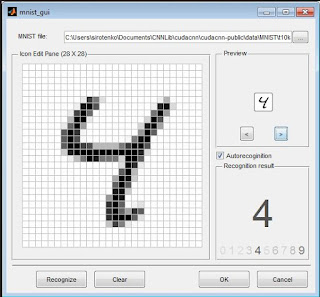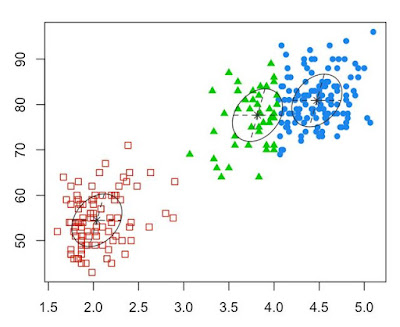# What is the Difference Between Supervised and Unsupervised Learning?

Supervised learning and unsupervised learning are the two branches of Machine Learning.
In my previous article Introduction to Machine Learning, I discussed the various categories and sub-categories of machine learning.

We would begin the discussion with a of Supervise Learning

Supervised Learning
This is a branch of machine learning that tries to find a relationship in a given training data set.
In supervised learning, the input consists of a set of input vector together with a corresponding target vectors.
In supervised learning, you have input variables (x) and out variable (y) and you have to use some algorithm to determine mapping function from the input to the output

y = f(x)

When this is done, then given a new input data, you can predict the output.

Why it is called ‘Supervised’
It is called supervised learning because the training data set is considered supervisory, that is it supervises the algorithm or controls the learning process. So if the algorithm, makes a wrong prediction, the training dataset corrects it. So the process is learning from the training/supervisory data set.

Supervised learning is divided into two categories: classification and regression.Figure 1: Digit recognition example of Classification

Classification: In classification, the objective is to assign each input vector to one of a given number of discrete categories. A typical example of classification is the image recognition problem. The input is a handwritten image made up of a grid of pixels, say 64 x 64. And the aim is to assign each image to one of 10 classes, 1 to 9.

Regression: In the case of regression the objective is to find the a relationship among the input variables. Regression analysis helps in understanding how the dependent variable changes with respect to the independent variables.

Unsupervised Learning
In unsupervised learning is a type of machine learning algorithm that draws inference from input datasets without corresponding labeled response. So here, you just have a set data values, say, only x, and you need to search for trends withing this dataset.
Unsupervised learning can further be divided into Clustering, Density Extimation and Dimensionality ReductionFigure 3: Clusters within the dataset
• In clustering, the goal is to find clusters or groups of similar examples withing the data.
• Density estimation has to do with identifying groups of data within the original dataset that belong to the same density distribution.
• Dimensionality Reduction reduces data from a higher dimensional space to lower dimension like 2 or 3 dimensions using the principal components.

Summary of the Difference between Supervised Learning and Unsupervised Learning is given in the table below:

 Supervised Learning Unsupervised Learning Input data is labelled Input data is unlabeled Uses training dataset Uses just input dataset Used for prediction Used for analysis Classification and regression Clustering, density estimation and dimensionality reduction

Other Machine Learning Resources